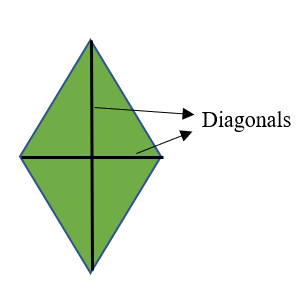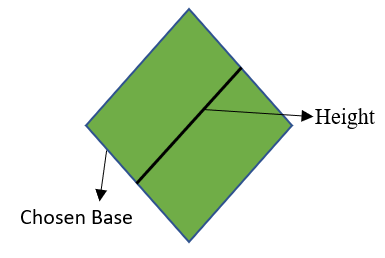# Area of Rhombus Formula

• Last Updated : 01 Feb, 2022

The concept of area, volume, length, etc. any measurement of a 2-Dimensional or 3-Dimensional figure/shape comes under the topic of mensuration. Mensuration is a branch of geometry that deals with the measurement of length, perimeter, area, volume, etc. of 2-Dimensional or 3-Dimensional figures. 2D shapes include circle, triangle, square, rectangle, rhombus, trapezium, etc. 3D figures include cubes, spheres, cylinders, cuboids, pyramids, etc. This article is about finding the area of the rhombus which is a 2D shape.

### Area of Rhombus

The rhombus has four sides that are equal in length and congruent. The area of a rhombus is the amount of space enclosed by a rhombus. The area of the rhombus can be calculated in three ways.

• Approach-1

When the lengths of diagonals are given then the area of the rhombus can be calculated by using the below formula,Area = (1/2) × length of diagonal1 × length of diagonal2

• Approach-2

The area of a rhombus is calculated using base and height where the base is the length of any side and height is the perpendicular distance from chosen base to the opposite side.The formula is given by:

Area of rhombus = length of base × height

• Approach-3

When the length of any side of the rhombus is given along with anyone interior angle then the area of a rhombus is calculated by the formula,

Area of rhombus = length of a side2 × sin(angle)

Note: The sine values for particular angles are,

### Sample Problems

Question 1: What is the area of the rhombus for which the length of diagonals is 4cm, 5cm.

Solution:

Given lengths of diagonals

D1 = 4cm

D2 = 5cm

Using approach 1,

Area of rhombus = (1/2) × 4 × 5

= (1/2) × 20

= 10

So the area of rhombus is 10cm2.

Question 2: Find the area of the rhombus where the length of diagonals is 7cm and 7cm.

Solution:

Given lengths of diagonals

D1 = 7cm

D2 = 7cm

Using approach 1,

Area of rhombus = (1/2) × 7 × 7

= (1/2) × 49

= 24.5

So the area of given rhombus is 24.5cm2.

Question 3: Find the area of a rhombus whose height is 4cm and base length is 6cm.

Solution:

Given,

Base length = 6cm

height = 4cm

Area of rhombus = base × height

= 6 × 4

= 24cm2

So area of given rhombus is 24cm2.

Question 4: Find the area of the rhombus where height is 6cm and base length is 8cm.

Solution:

Given,

Base length = 8cm

Height = 6cm

Using approach 2,

Area of rhombus = base × height

= 8 × 6

= 48cm2

So area of given rhombus is 24cm2.

Question 5: Find the area of the rhombus where the side of the rhombus is 5cm and one of the interior angles is 45°.

Solution:

Given,

Side length = 5cm

Interior angle = 45°

Using Approach 3,

Area of rhombus = 52 × sin(45°)

= 25 × (1/√2)

= 17.68 cm2

So the area of given rhombus is 17.68cm2

Question 6: Find the area of the rhombus where the length of a side of the rhombus is 4cm and one of the interior angles is 60°.

Solution:

Given,

Side length = 4cm

Interior angle = 60°

Using approach 3,

Area of rhombus = 42 × sin(60°)

= 16 × (1/(√3/2))

= 18.47 cm2 (approximately)

So the area of given rhombus is 18.47cm2

My Personal Notes arrow_drop_up